# Post Exam Python-Matplotlib tutorial¶

matplotlib is an excellent 2D (and 3D) python library that can be used to produce publication quality output from your data. The website https://matplotlib.org/ provides a complete resource for how to use matplotlib for your work. In particular if you click on an example plot in the gallery, https://matplotlib.org/gallery/index.html, the browser will display the code required to produce the plot. It is quite difficult to ask google "I would like my plot to look like this, and have these features, how do I do it?", however it is easy to browse through the gallery until you see the feature that you are interested in.

Unlike software like Excel in matplotlib you write code to determine the appearance of all aspects of your graph, you can recycle this code to easily create reproducable, consistent publication quality representations of your scientific data

Preparing the notebook for using matplotlib and numpy.

In :
%matplotlib inline
# this line is required for the plots to appear in the Jupyter cells, rather than launching the matplotlib GUI

import matplotlib

import numpy as np

import matplotlib.pyplot as plt

# Let printing work the same in Python 2 and 3
from __future__ import print_function

# notice two underscores _ either side of future


## Create some data for plotting examples.¶

In :
x=np.linspace(0,2*np.pi, 100)
y=np.cos(x)


### Generate the basic matplotlib 2D plot, figure() creates the space into which the plot will be added.¶

In :
plt.figure()
plt.plot(x,y)

Out:
[<matplotlib.lines.Line2D at 0x22c5ec95668>]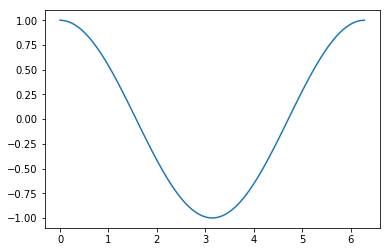In :
plt.figure()
plt.plot(x,y,'r+') # change the line style to red plusses highlights that we are dealing with a discrete set of points

Out:
[<matplotlib.lines.Line2D at 0x22c5ecf7a58>]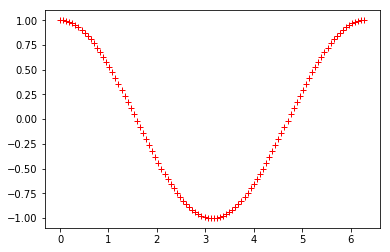### Within matplotlib.pyplot there are too many functions to describe here:¶

In :
print(dir(plt)) # matplotlib.pyplot is an extensive package

['Annotation', 'Arrow', 'Artist', 'AutoLocator', 'Axes', 'Button', 'Circle', 'Figure', 'FigureCanvasBase', 'FixedFormatter', 'FixedLocator', 'FormatStrFormatter', 'Formatter', 'FuncFormatter', 'GridSpec', 'IndexLocator', 'Line2D', 'LinearLocator', 'Locator', 'LogFormatter', 'LogFormatterExponent', 'LogFormatterMathtext', 'LogLocator', 'MaxNLocator', 'MultipleLocator', 'Normalize', 'NullFormatter', 'NullLocator', 'PolarAxes', 'Polygon', 'Rectangle', 'ScalarFormatter', 'Slider', 'Subplot', 'SubplotTool', 'Text', 'TickHelper', 'Widget', '_INSTALL_FIG_OBSERVER', '_IP_REGISTERED', '__builtins__', '__cached__', '__doc__', '__file__', '__loader__', '__name__', '__package__', '__spec__', '_auto_draw_if_interactive', '_autogen_docstring', '_backend_mod', '_backend_selection', '_hold_msg', '_imread', '_imsave', '_interactive_bk', '_pylab_helpers', '_setp', '_setup_pyplot_info_docstrings', '_show', '_string_to_bool', 'absolute_import', 'acorr', 'angle_spectrum', 'annotate', 'arrow', 'autoscale', 'autumn', 'axes', 'axhline', 'axhspan', 'axis', 'axvline', 'axvspan', 'bar', 'barbs', 'barh', 'bone', 'box', 'boxplot', 'broken_barh', 'cla', 'clabel', 'clf', 'clim', 'close', 'cm', 'cohere', 'colorbar', 'colormaps', 'colors', 'connect', 'contour', 'contourf', 'cool', 'copper', 'csd', 'cycler', 'dedent', 'delaxes', 'deprecated', 'disconnect', 'division', 'docstring', 'draw', 'draw_all', 'draw_if_interactive', 'errorbar', 'eventplot', 'figaspect', 'figimage', 'figlegend', 'fignum_exists', 'figtext', 'figure', 'fill', 'fill_between', 'fill_betweenx', 'findobj', 'flag', 'gca', 'gcf', 'gci', 'get', 'get_backend', 'get_cmap', 'get_current_fig_manager', 'get_figlabels', 'get_fignums', 'get_plot_commands', 'get_scale_docs', 'get_scale_names', 'getp', 'ginput', 'gray', 'grid', 'hexbin', 'hist', 'hist2d', 'hlines', 'hold', 'hot', 'hsv', 'imread', 'imsave', 'imshow', 'inferno', 'install_repl_displayhook', 'interactive', 'ioff', 'ion', 'is_numlike', 'ishold', 'isinteractive', 'jet', 'legend', 'locator_params', 'loglog', 'magma', 'magnitude_spectrum', 'margins', 'matplotlib', 'matshow', 'minorticks_off', 'minorticks_on', 'mlab', 'new_figure_manager', 'nipy_spectral', 'np', 'over', 'pause', 'pcolor', 'pcolormesh', 'phase_spectrum', 'pie', 'pink', 'plasma', 'plot', 'plot_date', 'plotfile', 'plotting', 'polar', 'print_function', 'prism', 'psd', 'pylab_setup', 'quiver', 'quiverkey', 'rc', 'rcParams', 'rcParamsDefault', 'rc_context', 'rcdefaults', 'register_cmap', 'rgrids', 'savefig', 'sca', 'scatter', 'sci', 'semilogx', 'semilogy', 'set_cmap', 'setp', 'show', 'silent_list', 'six', 'specgram', 'spectral', 'spring', 'spy', 'stackplot', 'stem', 'step', 'streamplot', 'style', 'subplot', 'subplot2grid', 'subplot_tool', 'subplots', 'subplots_adjust', 'summer', 'suptitle', 'switch_backend', 'sys', 'table', 'text', 'thetagrids', 'tick_params', 'ticklabel_format', 'tight_layout', 'time', 'title', 'tricontour', 'tricontourf', 'tripcolor', 'triplot', 'twinx', 'twiny', 'types', 'unicode_literals', 'uninstall_repl_displayhook', 'violinplot', 'viridis', 'vlines', 'waitforbuttonpress', 'warnings', 'winter', 'xcorr', 'xkcd', 'xlabel', 'xlim', 'xscale', 'xticks', 'ylabel', 'ylim', 'yscale', 'yticks']

In :
help(plt.plot) # the plot docstring gives a detailed set of instructions on the usasge

Help on function plot in module matplotlib.pyplot:

plot(*args, **kwargs)
Plot lines and/or markers to the
:class:~matplotlib.axes.Axes.  *args* is a variable length
argument, allowing for multiple *x*, *y* pairs with an
optional format string.  For example, each of the following is
legal::

plot(x, y)        # plot x and y using default line style and color
plot(x, y, 'bo')  # plot x and y using blue circle markers
plot(y)           # plot y using x as index array 0..N-1
plot(y, 'r+')     # ditto, but with red plusses

If *x* and/or *y* is 2-dimensional, then the corresponding columns
will be plotted.

If used with labeled data, make sure that the color spec is not
included as an element in data, as otherwise the last case
plot("v","r", data={"v":..., "r":...)
can be interpreted as the first case which would do plot(v, r)
using the default line style and color.

If not used with labeled data (i.e., without a data argument),
an arbitrary number of *x*, *y*, *fmt* groups can be specified, as in::

a.plot(x1, y1, 'g^', x2, y2, 'g-')

Return value is a list of lines that were added.

By default, each line is assigned a different style specified by a
'style cycle'.  To change this behavior, you can edit the
axes.prop_cycle rcParam.

The following format string characters are accepted to control
the line style or marker:

================    ===============================
character           description
================    ===============================
'-'             solid line style
'--'            dashed line style
'-.'            dash-dot line style
':'             dotted line style
'.'             point marker
','             pixel marker
'o'             circle marker
'v'             triangle_down marker
'^'             triangle_up marker
'<'             triangle_left marker
'>'             triangle_right marker
'1'             tri_down marker
'2'             tri_up marker
'3'             tri_left marker
'4'             tri_right marker
's'             square marker
'p'             pentagon marker
'*'             star marker
'h'             hexagon1 marker
'H'             hexagon2 marker
'+'             plus marker
'x'             x marker
'D'             diamond marker
'd'             thin_diamond marker
'|'             vline marker
'_'             hline marker
================    ===============================

The following color abbreviations are supported:

==========  ========
character   color
==========  ========
'b'         blue
'g'         green
'r'         red
'c'         cyan
'm'         magenta
'y'         yellow
'k'         black
'w'         white
==========  ========

In addition, you can specify colors in many weird and
wonderful ways, including full names ('green'), hex
strings ('#008000'), RGB or RGBA tuples ((0,1,0,1)) or
grayscale intensities as a string ('0.8').  Of these, the
string specifications can be used in place of a fmt group,
but the tuple forms can be used only as kwargs.

Line styles and colors are combined in a single format string, as in
'bo' for blue circles.

The *kwargs* can be used to set line properties (any property that has
a set_* method).  You can use this to set a line label (for auto
legends), linewidth, anitialising, marker face color, etc.  Here is an
example::

plot([1,2,3], [1,2,3], 'go-', label='line 1', linewidth=2)
plot([1,2,3], [1,4,9], 'rs',  label='line 2')
axis([0, 4, 0, 10])
legend()

If you make multiple lines with one plot command, the kwargs
apply to all those lines, e.g.::

plot(x1, y1, x2, y2, antialiased=False)

Neither line will be antialiased.

You do not need to use format strings, which are just
abbreviations.  All of the line properties can be controlled
by keyword arguments.  For example, you can set the color,
marker, linestyle, and markercolor with::

plot(x, y, color='green', linestyle='dashed', marker='o',
markerfacecolor='blue', markersize=12).

See :class:~matplotlib.lines.Line2D for details.

The kwargs are :class:~matplotlib.lines.Line2D properties:

agg_filter: a filter function, which takes a (m, n, 3) float array and a dpi value, and returns a (m, n, 3) array
alpha: float (0.0 transparent through 1.0 opaque)
animated: bool
antialiased or aa: [True | False]
clip_box: a ~.Bbox instance
clip_on: bool
clip_path: [(~matplotlib.path.Path, ~.Transform) | ~.Patch | None]
color or c: any matplotlib color
contains: a callable function
dash_capstyle: ['butt' | 'round' | 'projecting']
dash_joinstyle: ['miter' | 'round' | 'bevel']
dashes: sequence of on/off ink in points
drawstyle: ['default' | 'steps' | 'steps-pre' | 'steps-mid' | 'steps-post']
figure: a ~.Figure instance
fillstyle: ['full' | 'left' | 'right' | 'bottom' | 'top' | 'none']
gid: an id string
label: object
linestyle or ls: ['solid' | 'dashed', 'dashdot', 'dotted' | (offset, on-off-dash-seq) | '-' | '--' | '-.' | ':' | 'None' | ' ' | '']
linewidth or lw: float value in points
marker: :mod:A valid marker style <matplotlib.markers>
markeredgecolor or mec: any matplotlib color
markeredgewidth or mew: float value in points
markerfacecolor or mfc: any matplotlib color
markerfacecoloralt or mfcalt: any matplotlib color
markersize or ms: float
markevery: [None | int | length-2 tuple of int | slice | list/array of int | float | length-2 tuple of float]
path_effects: ~.AbstractPathEffect
picker: float distance in points or callable pick function fn(artist, event)
rasterized: bool or None
sketch_params: (scale: float, length: float, randomness: float)
snap: bool or None
solid_capstyle: ['butt' | 'round' |  'projecting']
solid_joinstyle: ['miter' | 'round' | 'bevel']
transform: a :class:matplotlib.transforms.Transform instance
url: a url string
visible: bool
xdata: 1D array
ydata: 1D array
zorder: float

kwargs *scalex* and *scaley*, if defined, are passed on to
:meth:~matplotlib.axes.Axes.autoscale_view to determine
whether the *x* and *y* axes are autoscaled; the default is
*True*.

.. note::
In addition to the above described arguments, this function can take a
**data** keyword argument. If such a **data** argument is given, the
following arguments are replaced by **data[<arg>]**:

* All arguments with the following names: 'x', 'y'.



plot(*args, **kwargs) refers to the functions arguments and keyword arguments. The order of the arguments in a python function determines how the argument is passed into the function i.e plot(x,y) will have x as the x-axis, plot(y,x) will have y as the x-axis. The kwargs can come in any order as they are recognised by the keyword i.e. label='my experimental data'.

## Returning to our plot:¶

The following code begins to show how much control you can have over the appearance of the plot, in particular note that LaTex math symbols have been used to label the xticks, and the ticks have been moved to user defined positions.

In :
z=np.sin(x)
plt.figure()
plt.plot(x,y, label=r'$cos(x)$')
plt.plot(x,z, label=r'$sin(x)$')# I have not specified the colour, but matplotlib will increment
#through a range as new plots are addded.
plt.legend(loc=1) # places the legend (created from the plot labels) in the upper-right
plt.title('My First Plot')
plt.xlabel(r'$\theta$') # the r tells python to read all characters, otherwise it would not read the \
plt.ylabel('y')
xmin,xmax=plt.xlim() # returns the current limits
plt.xlim(0,xmax*1.3) # sets new limits, makes some space on the right for the legend
plt.xticks((0,np.pi/2,np.pi,3*np.pi/2,2*np.pi),('0','$\pi/2$','$\pi$','$3\pi/2$','$2\pi$')) # Move the tick labels and use
#Latex commands for the labels
plt.tight_layout() #Ensures nothing overlaps
plt.show() # this is not needed in the notebook but is required from your code.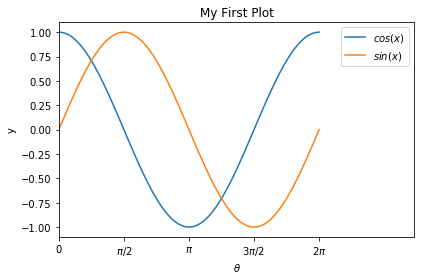In :
climate=np.loadtxt('https://climate.nasa.gov/system/internal_resources/details/original/647_Global_Temperature_Data_File.txt') # data downloaded from the NASA climate change website.

In :
np.shape(climate) # I want the first two columns in this array

Out:
(138, 3)
In :
year,tempchange=climate.transpose(),climate.transpose()
# by sepearting the variables with a comma we can assign both in a single line

In :
plt.figure()
plt.scatter(year,tempchange, label='NASA data')
plt.title('Nasa Climate Change data since 1880')
plt.xlabel('Year')
plt.ylabel('$\delta T$ from the 1951-1980 mean [C]')
plt.legend()
plt.show()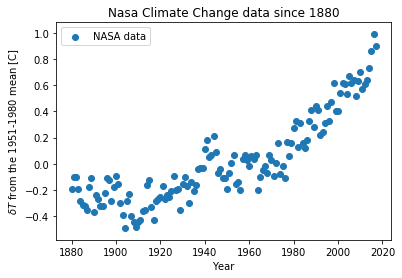As a quick look at how simple it can be to analyse your data with python the following histogram can be generated with a single additional line of code.

In :
plt.hist(tempchange)

Out:
(array([13., 25., 34., 22., 12.,  7., 10., 10.,  2.,  3.]),
array([-0.49 , -0.342, -0.194, -0.046,  0.102,  0.25 ,  0.398,  0.546,
0.694,  0.842,  0.99 ]),
<a list of 10 Patch objects>)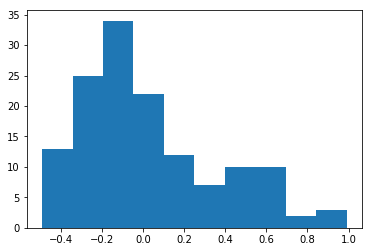## This notebook forms the basic introduction to plotting in python with matplotlib, next term we will expand on this with further topics:¶

• Adding multiple plots to a figure (subplots)
• Exploring different types of graph
• Imshow, Axes3D, plotting histograms
• Animate plots